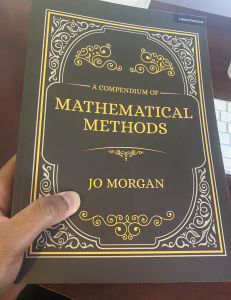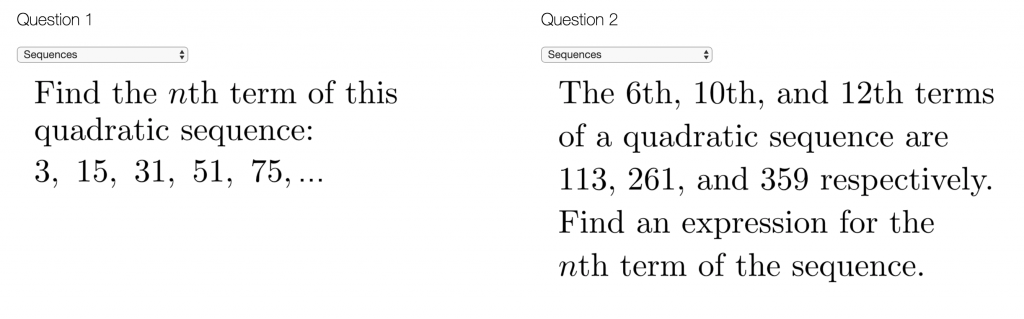# Let’s talk about methods (Part 1)

This is the first of a series of posts inspired by Jo Morgan’s rallying call to talk about methods. In this post, I’ll:

1. say a few words about Jo’s wonderful book, A Compendium of Mathematical Methods;
2. consider why I sometimes don’t teach the methods I like using; and
3. share a method that’s not in the Compendium.

In the subsequent posts in the series, I’ll share other methods (perhaps more fairly described as “tricks”) that I use regularly. While I came up with those independently, I’m sure others will have done so too—but I’ve never seen these tricks formally described anywhere online or in print.

## 1. A brief review of the CompendiumThe last couple of years have seen the launches of several high-profile books aimed at teachers (and maths teachers in particular). I sometimes wonder how there can be room for more, but I’ve found that these books have tended to carve their own niches well—and as such turned out to be worthwhile reading. One way in which the Compendium is delightfully different from other recent reads is that it’s not foremost a book on teaching maths or how students learn maths but a book where the maths itself takes centre stage.

The book contains plenty of methods I’d not come across before. For context, I have a maths degree, first volunteered as a classroom assistant in 2005, and became a teacher in 2008. As a maths lover, I can without a hint of exaggeration describe the experience of coming across certain new methods as joyous. If the book had included just one such method, I’d have been happy enough with it. In actuality, it had several such methods. I’d therefore strongly recommend the book to anyone that likes maths.

Finally, even where there were methods that I’d already come across, there were many, many tidbits I picked up from the book. Often, I realised that while I already knew the method, I had no idea about what the method was called or where it originated—until enlightened here by Jo. Many will know from Resourceaholic and from Jo’s workshops that she has long been interested in old textbooks and the Compendium gives us a glimpse at the breadth and depth of her familiarity with these. As such, it’s not just “a great book that could have been written by many others”; it’s a great book that could only have been written by its author.

## 2. Why I sometimes don’t teach the methods I’d use

I sometimes find myself not teaching methods that I like using myself. There are three main reasons for this. The first is that as a department, we might have agreed beforehand—for the sake of consistency—upon a certain different method for initial exposition. The second reason is that my preferred method is less versatile than the method I teach, though more efficient in specific cases. In an ideal world, I’d feel like I could teach the versatile method, and (a suitable amount of time later) teach the quicker method that applies in special cases. This leads me to the third reason: a (real or perceived) lack of time. Here are a couple of examples:

##### Example (i): Vertex of a quadratic

How would you find the coordinates of the vertex (in the below case, minimum point) of a quadratic? How would you teach this to a GCSE class? I suspect many teachers would reply completing the square to both questions.

I would absolutely teach the concept of completing the square because it is versatile and enlightening. Completing the square can not only be used to find the vertex, but also to solve quadratic equations. Further, developing an understanding of completing the square can help improve understanding of other characteristics of quadratics and it can be used to derive the quadratic formula itself.

That said, I might not always complete the square if faced with a specific question that only requires me to find the vertex—particularly where the quadratic is non-monic. Consider, for example, $$y= 5x^2+3x+11$$. Several months ago, I tweeted an approach to such a question (see below). I can typically do this kind of thing mentally, and therefore more quickly than I could do this by completing the square. By the way, this method is in the Compendium (see Method B in Chapter 16); only now do I realise this is well known as the “vertex formula.”

The method involves accepting that the $$x$$-coordinate will be $$\frac{-b}{2a}$$. It is not as versatile as completing the square, but it’s one I’d use whenever possible. Yet I’ve never taught this as a “go-to” method; indeed it’s an approach I’ve only shared (rightly or wrongly) with top-set GCSE classes and A-Level students in the past.

##### Example (ii): nth term of a quadratic sequence

I have flip-flopped between teaching the “simultaneous equations method” and the “residual method” (see Methods A and B in Chapter 13 if you have the book). In the former, we observe that if the $$n$$th term in $$an^2+bn+c$$, then the first term is $$a+b+c$$, the second is $$4a+2b+c$$, etc. In the latter, we spot that the quadratic sequence can be considered as the sum of a sequence of the form $$an^2$$ and a linear sequence.

Which of those methods would you use to answer the two questions below? While both work well for Question 1, this is not the case for Question 2. The simultaneous equations method extends naturally to Question 2, so it is more versatile than than the residual method, which is trickier to apply here. For this and other reasons, I now lean towards teaching the simultaneous equations method, and only later teach the residual method if time permits. If attempting questions myself, however, I would normally choose the residual method over the simultaneous equations method (wherever it can be used). I’m not sure I can put my finger on why, but I just prefer residual method!## 3. A method(?) that’s not in the Compendium

Sticking with the theme of quadratic sequences, there are of course other methods beyond the two noted above. Indeed, Jo describes six methods in her chapter on this topic! About a month ago, I tweeted an approach to finding the nth term of a quadratic sequence that’s not in the book (see the video in the tweet below). At the time, I thought of this as a possible basis for an investigation, rather than a method per se. Since then, however, having timed (🤓) myself having a go at a few questions, I’ve found the below approach (that I’ll call the “sequence of sequences method”) is usually at least as fast as the residual method for non-monic quadratic sequences and actually faster in the case of monic quadratics.

I still wouldn’t teach this (a) because I don’t consider it an enlightening method to a novice and (b) because it is not versatile (the method requires you to have the first few terms, so wouldn’t work for Question 2 above), but I reckon I’ll use it whenever possible because it is fast (at least for me, because my arithmetic is good and I’m quick with linear sequences.).